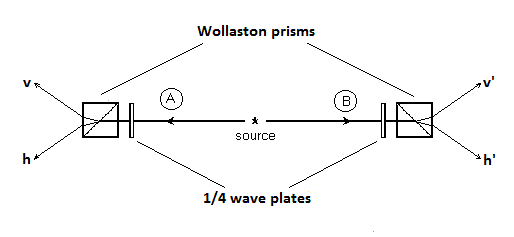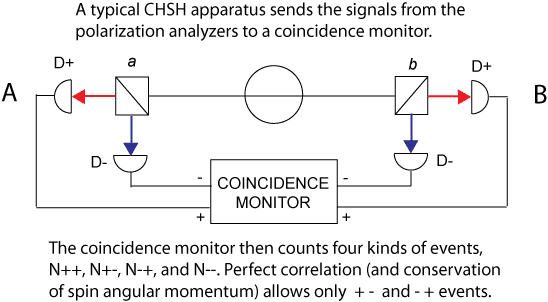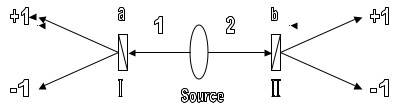# Entangled particles - Correlations

Let us say we have a EPR type experiment using entangled electrons. If the two observers have their detectors orientated so that they make correlating detections, then as I understand it, if one observer makes positive detections of electrons with "up spin" then the other observer has to make positive detections with "down spin". For example if Alice tests for up spin and Bob tests for down spin, then the results should look something like this:

Alice: 10010111011010001100
Bob_: 10010111011010001100

where 1 represents a positive detection. Now if both Alice and Bob test for up spin the results should look something like this:

Alice: 10010111011010001100
Bob_: 01011001010010110110

Now in a similar EPR experiment, but with entangled photons, does positive detection of photons with vertical polarisation by one observer correlate with positive detection of photons with horizontal polarisation by the other observer?

i.e if Alice test for vertical polarisation and Bob tests for horizontal polarisation the results should be something like this:

Alice: 10010111011010001100
Bob_: 10010111011010001100

but if they both test for vertical polarisation the results should look something like this:

Alice: 10010111011010001100
Bob_: 01011001010010110110

Is that correct? I am confused because some texts talk about correlating measurements agreeing when the polarisers have the same orientation and other texts say the positive correlations happen when the polarisers have opposite orientations. In other words, do entangled electrons have opposite spins and entangled photons have opposite polarisations?

K^2
No. You misunderstood entanglement completely. The states prior to measurement are 10+01. That means If Alice measures 1, she knows Bob will measure 0. If she measures 0, she knows Bob will measure 1. But prior to measurement, it's both 0 and 1.

No. You misunderstood entanglement completely.
Well I figured I probably did not completely understand entanglement. Hence the question! :tongue:

The states prior to measurement are 10+01.
Ok, as I understand it, prior to measurement the particle is neither up spin or down spin but a superimposition of both states. Take a look at this youtube video http://www.youtube.com/watch?v=9lOWZ0Wv218&feature=related starting at 1:53 (to skip the bloodshot eyeballs). It shows the two entangled electrons "flip flopping" between up and down spin, but at any given time they have opposite spin. Now would I be correct in thinking that is not entirely correct? I suppose technically, the video is wrong in that we can say nothing about the location of the photons before measurement, but that is perhaps too picky and makes "visualisation" almost impossible. Before measurement a given electron is neither up or down at a given time. Is that right?

That means If Alice measures 1, she knows Bob will measure 0. If she measures 0, she knows Bob will measure 1. But prior to measurement, it's both 0 and 1.
If Alice measures 1, she knows Bob will measure 0 if (and only if) she has absolute and instantaneous knowledge that Bob's measuring device has the same orientation as her own measuring device. If she has absolute knowledge that Bob's measuring device has "the opposite orientation" then she knows there will be no correlation between her measurement and Bob's. So in this case if she measures the vertical polarisation of a photon and gets a positive detection, she knows there is a 50/50 chance of Bob getting a correlating measurement. Is that right?

K^2
It's not flip-flopping between two states. It's both at once. But I guess it's hard to show on the video.

To put it more precisely, a particle has a definite state. That state does not happen to correspond to the state "up" nor the state "down", but it has projections onto both of these states, so if you measure it, you have chance to measure "up" and chance to measure "down". (Maybe you got that bit, this is just for sake of clarity.)

As far as Alice and Bob... Let me put it this way. After Alice makes her measurement, she knows the state of Bob's particle. Yes, if Bob happens to have chosen a completely different set of directions for polarization or spin up/down measurement, she cannot predict the outcome of the measurement. At best, she may be able to give probabilities of either outcome. Assuming she knows the orientation of Bob's measurement device, of course.

If you want to understand this a bit better, you might want to look a bit more into the algebra of SU2 space. Take a look at this article. If it doesn't scroll you to the right place, you are looking for a section titled "Mathematical Formulation of Spin[/url]. It describes algebra of Sx, Sy, and Sz operators. The three of them form a vector operator S[/s], which you can use to easily find a spin operator in arbitrary direction. Sn = Sn, where n is the vector of the axis along which you want to make a spin measurement.

If you want to understand this a bit better, you might want to look a bit more into the algebra of SU2 space. Take a look at this article. If it doesn't scroll you to the right place, you are looking for a section titled "Mathematical Formulation of Spin[/url]. It describes algebra of Sx, Sy, and Sz operators. The three of them form a vector operator S[/s], which you can use to easily find a spin operator in arbitrary direction. Sn = Sn, where n is the vector of the axis along which you want to make a spin measurement.
Thanks for your response. It will take me a while to understand the new formalisms and symbols there and I can perhaps learn easier if I can be given some practical examples to relate to.

First, could you confirm that for a pair of entangled photons created in a down converter, can we think of the photons going in opposite directions having opposite polarisation? Is this polarisation circular or linear?

Secondly, could you have a look at this ideal EPR set up.Now lets say the photon going to the left towards Alice (A) has left hand circular polarisation and is transformed by the quarter wave plate into a vertical linearly polarised photon. This photon then passes through the Wollaston prism and follows the v path to a detector registering a positive detection. The other entangled photon of the pair going towards Bob (B) has right handed circular polarisation (presumably). On reaching the quarter wave plate it is transformed into horizontal linearly polarised light and goes to the h' detector of Bob. Now lets say Alices primary detector is v and Bob's primary detector is v' then the results of a ten photon pair series might look like this:

v = 1001011100
v' = 0110100011

So we have a 100% correlation here. Every "1" received by Alice corresponds to a "0" received by Bob and vice versa.

Now if we happen to be recording the horizontal channels at the same time, the results of those two channels should like this:

h = 0110100011
h' = 1001011100

I am assuming that is what you would record because every time we detect a photon on the v channel we should get no detection on the h channel and vice versa. We either get a positive detection at v or at h but not both simultaneously.

Now the problem is this. If Alice make detections on her v channel and Bob makes detections on his h' channel, then the results look like this:

v = 1001011100
h' = 1001011100

but this is not what QM predicts. If Alice and Bob have different orientations for their detectors (Alice is measuring vertical polarisation and Bob is measuring horizontal polarisation) then there should be only be a random correlation and not 100% 1 for 1 agreement. What is it that I am misunderstanding here? Is the experimental setup wrong? Are my assumptions wrong? Please correct my mistakes if you can and describe a working model.

A. Neumaier
If Alice and Bob have different orientations for their detectors (Alice is measuring vertical polarisation and Bob is measuring horizontal polarisation) then there should be only be a random correlation and not 100% 1 for 1 agreement. What is it that I am misunderstanding here? Is the experimental setup wrong? Are my assumptions wrong?

Your first remark in the quoted text is correct. But the general assumption is that Alice and Bob measure the same observable, which conflicts with the assumption you made.

Your first remark in the quoted text is correct. But the general assumption is that Alice and Bob measure the same observable, which conflicts with the assumption you made.
Could you elaborate on my mistaken assumptions (I am sure there some, but not exactly sure where).

For example if the following observations are made on the v channels:

v = 1001011100
v' = 0110100011

can you give a similar example of what should be recorded on the h channels?

Also, it might well be that the experiment has to be modified. For example do I need to place regular polarisers after the 1/4 wave plates so that if Bob has his polariser orientated vertically he can only detect vertically polarised photons?

I think I understand that if an observation is made of say the vertical polarisation of the a photon, then the horizontal component is indeterminate (correct word?). In other words we can not have certain knowledge of both complementary observables of photon by measuring one component is entangled photon and the other component in its entangled partner. Correct?

The set up in the earlier post possibly does not work because it seems to set out to acquire complete knowledge of complementary observables by measuring the v and h polarisations of both entangled particles. However, I have seen examples in the literature that seem to be set up like this with dual channel analysers and maybe I misunderstanding something. For example:and figures 17 and 18 of this paper by Alain Aspect himself: http://arxiv.org/ftp/quant-ph/papers/0402/0402001.pdf

A. Neumaier
Could you elaborate on my mistaken assumptions (I am sure there some, but not exactly sure where).

I am not interested in discussing particular experiments or arrangements. I was just pointing out that you can't expect to understand an experiment where Alice and Bob measure the same observable by substituting two different observables (horizontal and vertical polarization).

zonde
Gold Member
I think I understand that if an observation is made of say the vertical polarisation of the a photon, then the horizontal component is indeterminate (correct word?). In other words we can not have certain knowledge of both complementary observables of photon by measuring one component is entangled photon and the other component in its entangled partner. Correct?
Incorrect. Measurements of horizontal and vertical polarization are commuting i.e. if you take measurement of horizontal polarization for Alice then you will have perfect agreement with measurement of vertical polarization for Bob and perfect disagreement with measurement of horizontal polarization for Bob.

In order to have no correlation for two measurements you have to measure one photon in H/V basis and the other in +45°/-45° basis. Measurements are complimentary if two bases differ by 45°.

Incorrect. Measurements of horizontal and vertical polarization are commuting i.e. if you take measurement of horizontal polarization for Alice then you will have perfect agreement with measurement of vertical polarization for Bob and perfect disagreement with measurement of horizontal polarization for Bob.

In order to have no correlation for two measurements you have to measure one photon in H/V basis and the other in +45°/-45° basis. Measurements are complimentary if two bases differ by 45°.

Could someone confirm or deny something here. Wikipedia states in its article on http://en.wikipedia.org/wiki/Spontaneous_parametric_down-conversion" [Broken] that
However, if one of the pair (the "signal") is detected at any time then we know its partner (the "idler") is present. Either signal and idler photons share the same polarisation (type I) or they have perpendicular polarisations (type II). The output of a type I down converter is a squeezed vacuum that contains only even photon number terms. The output of the type II down converter is a two-mode squeezed vacuum.

This means that if the source of the entangled photons is type II then the statement by zonde is correct, but if the source is type I then zonde is incorrect and if you take measurement of horizontal polarization for Alice then you will have perfect agreement with measurement of horizontal polarization for Bob and perfect disagreement with measurement of vertical polarization for Bob. Is this a correct interpretation? If so it seams we should be more careful to mention the type (I or II) of the source, which is rarely done in these discussions.

Last edited by a moderator:
DrChinese
Gold Member
This means that if the source of the entangled photons is type II then the statement by zonde is correct, but if the source is type I then zonde is incorrect and if you take measurement of horizontal polarization for Alice then you will have perfect agreement with measurement of horizontal polarization for Bob and perfect disagreement with measurement of vertical polarization for Bob. Is this a correct interpretation? If so it seams we should be more careful to mention the type (I or II) of the source, which is rarely done in these discussions.

Zonde was correct, he was simply assuming we knew which Type. You are correct that the Type can be relevant. I always use Type I in my examples because it makes it easier to follow when you assume both photons are of the same polarization.

A quick summary for those who are interested:

Type I generally produces entangled photon pairs that have identical polarization. It takes 2 PDC crystals - perpendicular to each other - to achieve this.

Type II generally produces entangled photon pairs that have perpendicular (crossed or orthogonal) polarization. It takes 1 PDC crystal to achieve this.

Type I generally produces entangled photon pairs that have identical polarization. It takes 2 PDC crystals - perpendicular to each other - to achieve this.

Type II generally produces entangled photon pairs that have perpendicular (crossed or orthogonal) polarization. It takes 1 PDC crystal to achieve this.
Thank you for the additional information DrC. Is it possible to covert a type II source into a type I source by using a 1/2 wave plate to invert the polarisation of one photon relative to the other, or would this interfere with the entanglement and cause de-coherence?

DrChinese